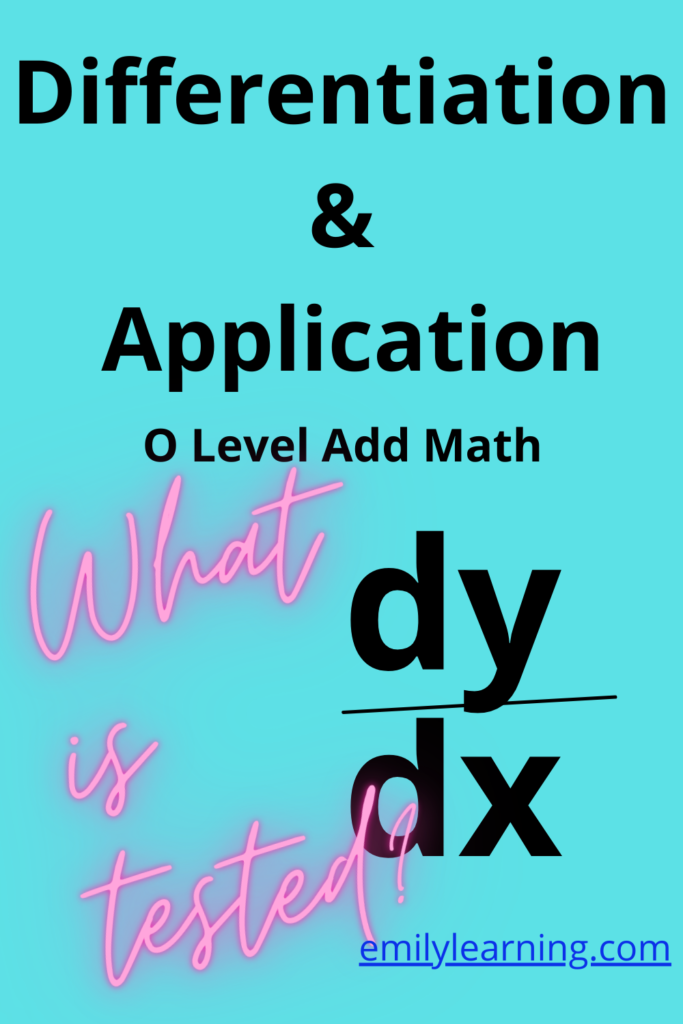# What you need to know for Differentiation and its application tested in O Level Additional MathematicsDifferentiation is a big topic in O Level Additional Mathematics. If you are using the textbook, you will notice the many chapters (and pages) devoted to just this entire topic. You are also required to know concepts from previous topics such as trigonometry, exponentials, and logarithms as you are required to do some manipulation of trigonometric, exponential, and logarithmic functions occasionally.

The entire differentiation is a big topic, but no fear! Once you master it, it’s easy to score. The questions also quite predictable, so you can basically for the steps for each type of question and score.

Here’s what’s tested in differentiation:

• Meaning of differentiation
• Using first principe to derive dy/dx for simple algebraic expressions
• finding first derivative (dy/dx), second derivatve (d2y/dx2) and so on
• Applications of chain rule, product rule, and quotient rule to differentiation
• differentiation of :
• algebraic functions
• trigonometric functions
• exponents
• logarithmic functions (in particular functions involving natural logarithm or ln
• relationship between dy/dx and increasing or decreasing function
• rate of change and chain rule
• stationary points and nature of stationary points
• problems involving maximum and minimum

## Want to learn O Level Additional Math on-demand?

Check out our on-demand O Level Additional Math course here.

error: Content is protected !!